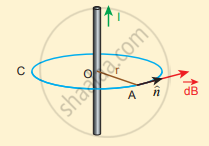Tamil Nadu Board of Secondary EducationHSC Science Class 12th

# Find the magnetic field due to a long straight conductor using Ampere’s circuital law. - Physics

Numerical

Find the magnetic field due to a long straight conductor using Ampere’s circuital law.

#### Solution

Consider a straight conductor of infinite length carrying current I and the direction of magnetic field lines. Since the wire is geometrically cylindrical in shape C and symmetrical about its axis, we construct an Amperian loop in the form of a circular shape at a distance r from the centre of the conductor. From the Ampere’s law, we getAmpèrian loop for current carrying straight wire

oint_"C" vec"B"*"d"vec"l" = mu_0 "I"

Where dl is the line element along the amperian loop (tangent to the circular loop). Hence, the angle between the magnetic field vector and line element is zero. Therefore,

oint_"C" "B"*"dl" = mu_0 "I"

where I is the current enclosed by the Amperian loop. Due to the symmetry, the magnitude of the magnetic field is uniform over the Amperian loop, we can take B out of the integration.

"B"oint_"C" "dl" = mu_0"I"

For a circular loop, the circumference is 2πr, which implies,

"B"int_hat"n"^(2pi"r") "dl" = mu_0 "I"

vec"B"*2pi"r" = mmu_0"I"

=> vec"B" = (mu_0"I")/(2pi"r")

In vector form, the magnetic field is

vec"B" = (mu_0"I")/(2pi"r")hat"n"

Where hat"n" is the unit vector along the tangent to the Amperian loop. This perfectly agrees with the result obtained from Biot-Savarf s law as given in equation

vec"B" = (mu_0"I")/(2pi"a") hat"n"

Concept: Ampere’s Circuital Law
Is there an error in this question or solution?
Chapter 3: Magnetism and magnetic effects of electric current - Evaluation [Page 192]

#### APPEARS IN

Tamil Nadu Board Samacheer Kalvi Class 12th Physics Volume 1 and 2 Answers Guide
Chapter 3 Magnetism and magnetic effects of electric current
Evaluation | Q III. 7. | Page 192
Share